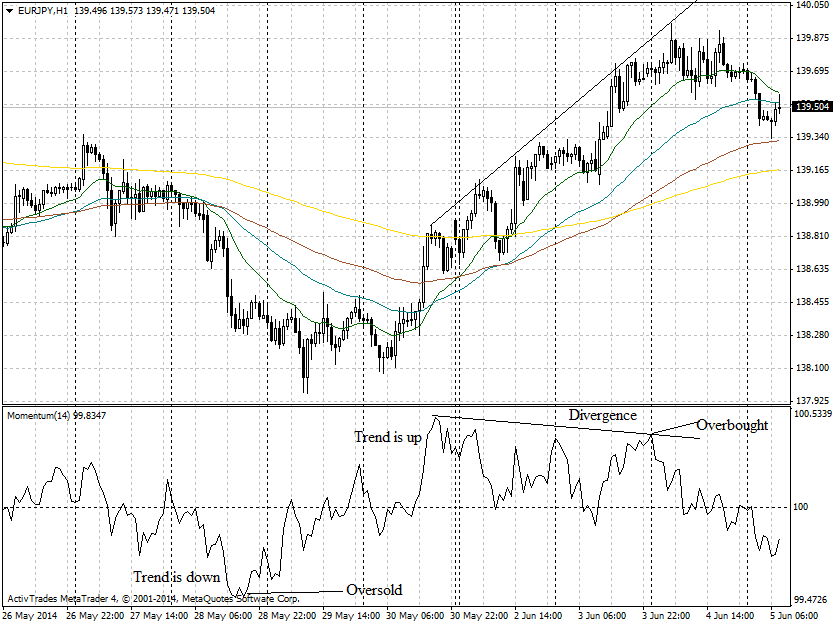# forex software

Create and Test Forex Strategies

forex software

trading:momentum-indicator

# Momentum indicator

Momentum is showing the difference between current closing price and the close N periods ago. This indicator measures the acceleration of the price move and could be calculated by the following formula:

Momentum = C(t) - C(t-n), where

C(t) is the latest closing price

C(t-n) is the closing price “n” ago

The idea is that if the price is higher than “n” periods ago it will continue to move up and vice versa. Momentum indicator is generating the following signals:

• Values above the Zero line - trend is up
• Values below Zero line - trend is down
• Extreme levels indicate overbought/oversold market conditions
• Divergence of the price and indicator charts